# fplot3

Plot 3-D parametric curve

## Syntax

fplot3(xt,yt,zt)
fplot3(xt,yt,zt,[tmin tmax])
fplot3(___,LineSpec)
fplot3(___,Name,Value)
fplot3(ax,___)
fp = fplot3(___)

## Description

example

fplot3(xt,yt,zt) plots the parametric curve xt = x(t), yt = y(t), and zt = z(t) over the default interval –5 < t < 5.

example

fplot3(xt,yt,zt,[tmin tmax]) plots xt = x(t), yt = y(t), and zt = z(t) over the interval tmin < t < tmax.

example

fplot3(___,LineSpec) uses LineSpec to set the line style, marker symbol, and line color.

example

fplot3(___,Name,Value) specifies line properties using one or more Name,Value pair arguments. Use this option with any of the input argument combinations in the previous syntaxes. Name,Value pair settings apply to all the lines plotted. To set options for individual lines, use the objects returned by fplot3.
fplot3(ax,___) plots into the axes object ax instead of the current axes gca.

example

fp = fplot3(___) returns a parameterized function line object. Use the object to query and modify properties of a specific parameterized line. For details, see ParameterizedFunctionLine Properties.

## Examples

### Plot 3-D Parametric Line

Plot the 3-D parametric line

$\begin{array}{c}x=\mathrm{sin}\left(t\right)\\ y=\mathrm{cos}\left(t\right)\\ z=t\end{array}$

over the default parameter range [-5 5].

syms t xt = sin(t); yt = cos(t); zt = t; fplot3(xt,yt,zt)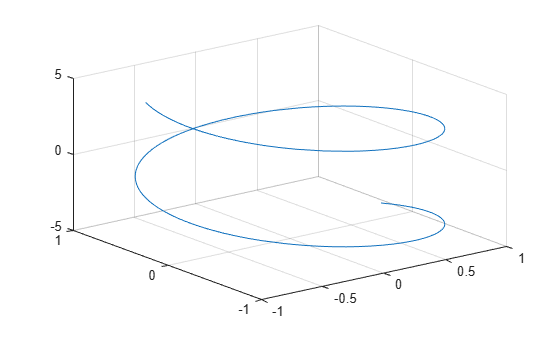### Specify Parameter Range

Plot the parametric line

$\begin{array}{c}x={e}^{-t/10}\mathrm{sin}\left(5t\right)\\ y={e}^{-t/10}\mathrm{cos}\left(5t\right)\\ z=t\end{array}$

over the parameter range [-10 10] by specifying the fourth argument of fplot3.

syms t xt = exp(-t/10).*sin(5*t); yt = exp(-t/10).*cos(5*t); zt = t; fplot3(xt,yt,zt,[-10 10])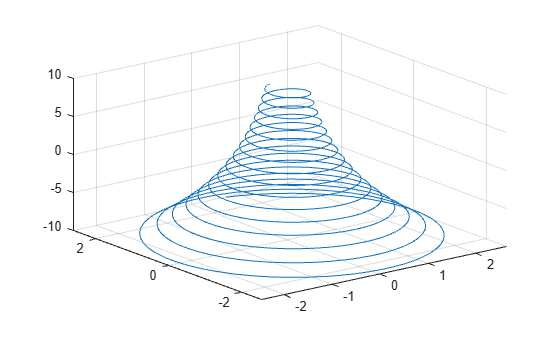### Change Line Properties and Display Markers

Plot the same 3-D parametric curve three times over different intervals of the parameter. For the first curve, use a linewidth of 2. For the second, specify a dashed red line style with circle markers. For the third, specify a cyan, dash-dot line style with asterisk markers.

syms t fplot3(sin(t), cos(t), t, [0 2*pi], 'LineWidth', 2) hold on fplot3(sin(t), cos(t), t, [2*pi 4*pi], '--or') fplot3(sin(t), cos(t), t, [4*pi 6*pi], '-.*c')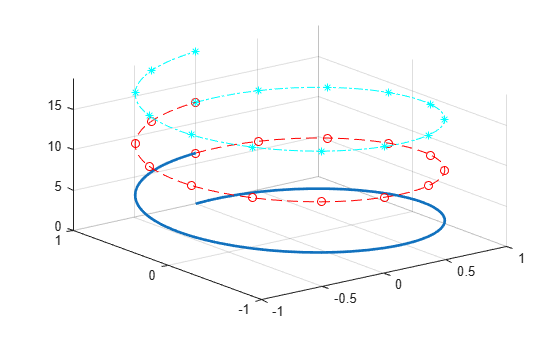### Plot 3-D Parametric Line Using Symbolic Functions

Plot the 3-D parametric line

$\begin{array}{c}x\left(t\right)=\mathrm{sin}\left(t\right)\\ y\left(t\right)=\mathrm{cos}\left(t\right)\\ z\left(t\right)=\mathrm{cos}\left(2t\right).\end{array}$

syms x(t) y(t) z(t) x(t) = sin(t); y(t) = cos(t); z(t) = cos(2*t); fplot3(x,y,z)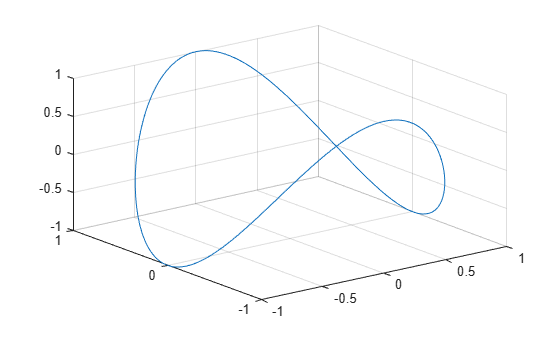### Plot Multiple Lines on Same Figure

Plot multiple lines either by passing the inputs as a vector or by using hold on to successively plot on the same figure. If you specify LineSpec and Name-Value arguments, they apply to all lines. To set options for individual lines, use the function handles returned by fplot3.

Divide a figure into two subplots using subplot. On the first subplot, plot two parameterized lines using vector input. On the second subplot, plot the same lines using hold on.

syms t subplot(2,1,1) fplot3([t -t], t, [t -t]) title('Multiple Lines Using Vector Inputs') subplot(2,1,2) fplot3(t, t, t) hold on fplot3(-t, t, -t) title('Multiple Lines Using Hold On Command') hold off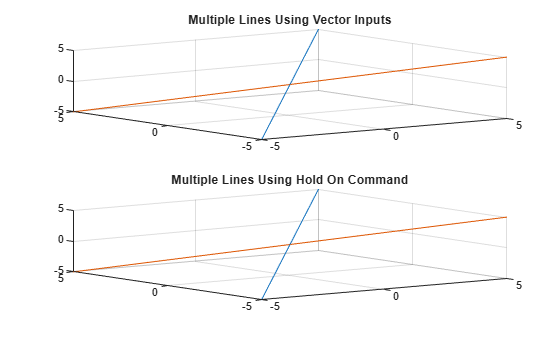### Modify 3-D Parametric Line After Creation

Plot the parametric line

$\begin{array}{l}x={e}^{-|t|/10}\mathrm{sin}\left(5|t|\right)\\ y={e}^{-|t|/10}\mathrm{cos}\left(5|t|\right)\\ z=t.\end{array}$

Provide an output to make fplot return the plot object.

syms t xt = exp(-abs(t)/10).*sin(5*abs(t)); yt = exp(-abs(t)/10).*cos(5*abs(t)); zt = t; fp = fplot3(xt,yt,zt)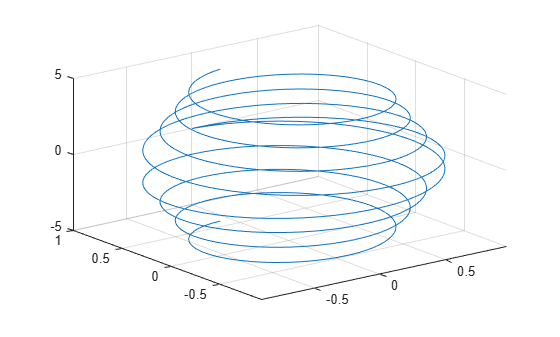fp = ParameterizedFunctionLine with properties: XFunction: [1x1 sym] YFunction: [1x1 sym] ZFunction: [1x1 sym] Color: [0 0.4470 0.7410] LineStyle: '-' LineWidth: 0.5000 Show all properties 

Change the range of parameter values to [-10 10] and the line color to red by using the TRange and Color properties of fp respectively.

fp.TRange = [-10 10]; fp.Color = 'r';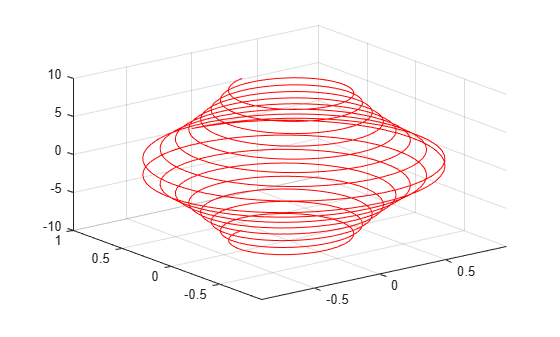### Add Title and Axis Labels and Format Ticks

For $t$ values in the range $-2\pi$ to $2\pi$, plot the parametric line

$\begin{array}{c}x=t\\ y=t/2\\ z=\mathrm{sin}\left(6t\right).\end{array}$

Add a title and axis labels. Create the x-axis ticks by spanning the x-axis limits at intervals of pi/2. Display these ticks by using the XTick property. Create x-axis labels by using arrayfun to apply texlabel to S. Display these labels by using the XTickLabel property. Repeat these steps for the y-axis.

To use LaTeX in plots, see latex.

syms t xt = t; yt = t/2; zt = sin(6*t); fplot3(xt,yt,zt,[-2*pi 2*pi],'MeshDensity',30) view(52.5,30) xlabel('x') ylabel('y') title('x=t, y=t/2, z=sin(6t) for -2\pi < t < 2\pi') ax = gca; S = sym(ax.XLim(1):pi/2:ax.XLim(2)); ax.XTick = double(S); ax.XTickLabel = arrayfun(@texlabel, S, 'UniformOutput', false); S = sym(ax.YLim(1):pi/2:ax.YLim(2)); ax.YTick = double(S); ax.YTickLabel = arrayfun(@texlabel, S, 'UniformOutput', false);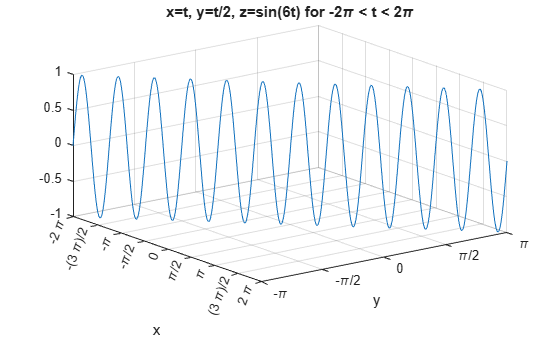### Create Animations

Create animations by changing the displayed expression using the XFunction, YFunction, and ZFunction properties and then by using drawnow to update the plot. To export to GIF, see imwrite.

By varying the variable i from 0 to 4π, animate the parametric curve

$\begin{array}{l}x=t+\mathrm{sin}\left(40t\right)\\ y=-t+\mathrm{cos}\left(40t\right)\\ z=\mathrm{sin}\left(t+i\right).\end{array}$

To play the animation, click the image.

syms t fp = fplot3(t+sin(40*t),-t+cos(40*t), sin(t)); for i=0:pi/10:4*pi fp.ZFunction = sin(t+i); drawnow end## Input Arguments

collapse all

Parametric input for x-axis, specified as a symbolic expression or function. fplot3 uses symvar to find the parameter.

Parametric input for y-axis, specified as a symbolic expression or function. fplot3 uses symvar to find the parameter.

Parametric input for z-axis, specified as a symbolic expression or function. fplot3 uses symvar to find the parameter.

Range of values of parameter, specified as a vector of two numbers. The default range is [-5 5].

Axes object. If you do not specify an axes object, then fplot3 uses the current axes.

Line style, marker, and color, specified as a character vector or string containing symbols. The symbols can appear in any order. You do not need to specify all three characteristics (line style, marker, and color). For example, if you omit the line style and specify the marker, then the plot shows only the marker and no line.

Example: '--or' is a red dashed line with circle markers

Line StyleDescription
-Solid line
--Dashed line
:Dotted line
-.Dash-dot line
MarkerDescription
'o'Circle
'+'Plus sign
'*'Asterisk
'.'Point
'x'Cross
'_'Horizontal line
'|'Vertical line
's'Square
'd'Diamond
'^'Upward-pointing triangle
'v'Downward-pointing triangle
'>'Right-pointing triangle
'<'Left-pointing triangle
'p'Pentagram
'h'Hexagram
ColorDescription

y

yellow

m

magenta

c

cyan

r

red

g

green

b

blue

w

white

k

black

### Name-Value Pair Arguments

Specify optional comma-separated pairs of Name,Value arguments. Name is the argument name and Value is the corresponding value. Name must appear inside quotes. You can specify several name and value pair arguments in any order as Name1,Value1,...,NameN,ValueN.

Example: 'Marker','o','MarkerFaceColor','red'

The properties listed here are only a subset. For a complete list, see ParameterizedFunctionLine Properties.

Number of evaluation points, specified as a number. The default is 23. Because fplot3 uses adaptive evaluation, the actual number of evaluation points is greater.

Line color, specified as an RGB triplet, a hexadecimal color code, a color name, or a short name.

For a custom color, specify an RGB triplet or a hexadecimal color code.

• An RGB triplet is a three-element row vector whose elements specify the intensities of the red, green, and blue components of the color. The intensities must be in the range [0,1]; for example, [0.4 0.6 0.7].

• A hexadecimal color code is a character vector or a string scalar that starts with a hash symbol (#) followed by three or six hexadecimal digits, which can range from 0 to F. The values are not case sensitive. Thus, the color codes '#FF8800', '#ff8800', '#F80', and '#f80' are equivalent.

Alternatively, you can specify some common colors by name. This table lists the named color options, the equivalent RGB triplets, and hexadecimal color codes.

Color NameShort NameRGB TripletHexadecimal Color CodeAppearance
'red''r'[1 0 0]'#FF0000''green''g'[0 1 0]'#00FF00''blue''b'[0 0 1]'#0000FF''cyan' 'c'[0 1 1]'#00FFFF''magenta''m'[1 0 1]'#FF00FF''yellow''y'[1 1 0]'#FFFF00''black''k'[0 0 0]'#000000''white''w'[1 1 1]'#FFFFFF'Here are the RGB triplets and hexadecimal color codes for the default colors MATLAB® uses in many types of plots.

[0 0.4470 0.7410]'#0072BD'[0.8500 0.3250 0.0980]'#D95319'[0.9290 0.6940 0.1250]'#EDB120'[0.4940 0.1840 0.5560]'#7E2F8E'[0.4660 0.6740 0.1880]'#77AC30'[0.3010 0.7450 0.9330]'#4DBEEE'[0.6350 0.0780 0.1840]'#A2142F'Example: 'blue'

Example: [0 0 1]

Example: '#0000FF'

Line style, specified as one of the options listed in this table.

Line StyleDescriptionResulting Line
'-'Solid line'--'Dashed line':'Dotted line'-.'Dash-dotted line'none'No lineNo line

Line width, specified as a positive value in points, where 1 point = 1/72 of an inch. If the line has markers, then the line width also affects the marker edges.

The line width cannot be thinner than the width of a pixel. If you set the line width to a value that is less than the width of a pixel on your system, the line displays as one pixel wide.

Marker symbol, specified as one of the values listed in this table. By default, the object does not display markers. Specifying a marker symbol adds markers at each data point or vertex.

ValueDescription
'o'Circle
'+'Plus sign
'*'Asterisk
'.'Point
'x'Cross
'_'Horizontal line
'|'Vertical line
'square' or 's'Square
'diamond' or 'd'Diamond
'^'Upward-pointing triangle
'v'Downward-pointing triangle
'>'Right-pointing triangle
'<'Left-pointing triangle
'pentagram' or 'p'Five-pointed star (pentagram)
'hexagram' or 'h'Six-pointed star (hexagram)
'none'No markers

Marker outline color, specified as 'auto', an RGB triplet, a hexadecimal color code, a color name, or a short name. The default value of 'auto' uses the same color as the Color property.

For a custom color, specify an RGB triplet or a hexadecimal color code.

• An RGB triplet is a three-element row vector whose elements specify the intensities of the red, green, and blue components of the color. The intensities must be in the range [0,1]; for example, [0.4 0.6 0.7].

• A hexadecimal color code is a character vector or a string scalar that starts with a hash symbol (#) followed by three or six hexadecimal digits, which can range from 0 to F. The values are not case sensitive. Thus, the color codes '#FF8800', '#ff8800', '#F80', and '#f80' are equivalent.

Alternatively, you can specify some common colors by name. This table lists the named color options, the equivalent RGB triplets, and hexadecimal color codes.

Color NameShort NameRGB TripletHexadecimal Color CodeAppearance
'red''r'[1 0 0]'#FF0000''green''g'[0 1 0]'#00FF00''blue''b'[0 0 1]'#0000FF''cyan' 'c'[0 1 1]'#00FFFF''magenta''m'[1 0 1]'#FF00FF''yellow''y'[1 1 0]'#FFFF00''black''k'[0 0 0]'#000000''white''w'[1 1 1]'#FFFFFF''none'Not applicableNot applicableNot applicableNo color

Here are the RGB triplets and hexadecimal color codes for the default colors MATLAB uses in many types of plots.

[0 0.4470 0.7410]'#0072BD'[0.8500 0.3250 0.0980]'#D95319'[0.9290 0.6940 0.1250]'#EDB120'[0.4940 0.1840 0.5560]'#7E2F8E'[0.4660 0.6740 0.1880]'#77AC30'[0.3010 0.7450 0.9330]'#4DBEEE'[0.6350 0.0780 0.1840]'#A2142F'Marker fill color, specified as 'auto', an RGB triplet, a hexadecimal color code, a color name, or a short name. The 'auto' value uses the same color as the MarkerEdgeColor property.

For a custom color, specify an RGB triplet or a hexadecimal color code.

• An RGB triplet is a three-element row vector whose elements specify the intensities of the red, green, and blue components of the color. The intensities must be in the range [0,1]; for example, [0.4 0.6 0.7].

• A hexadecimal color code is a character vector or a string scalar that starts with a hash symbol (#) followed by three or six hexadecimal digits, which can range from 0 to F. The values are not case sensitive. Thus, the color codes '#FF8800', '#ff8800', '#F80', and '#f80' are equivalent.

Alternatively, you can specify some common colors by name. This table lists the named color options, the equivalent RGB triplets, and hexadecimal color codes.

Color NameShort NameRGB TripletHexadecimal Color CodeAppearance
'red''r'[1 0 0]'#FF0000''green''g'[0 1 0]'#00FF00''blue''b'[0 0 1]'#0000FF''cyan' 'c'[0 1 1]'#00FFFF''magenta''m'[1 0 1]'#FF00FF''yellow''y'[1 1 0]'#FFFF00''black''k'[0 0 0]'#000000''white''w'[1 1 1]'#FFFFFF''none'Not applicableNot applicableNot applicableNo color

Here are the RGB triplets and hexadecimal color codes for the default colors MATLAB uses in many types of plots.

[0 0.4470 0.7410]'#0072BD'[0.8500 0.3250 0.0980]'#D95319'[0.9290 0.6940 0.1250]'#EDB120'[0.4940 0.1840 0.5560]'#7E2F8E'[0.4660 0.6740 0.1880]'#77AC30'[0.3010 0.7450 0.9330]'#4DBEEE'[0.6350 0.0780 0.1840]'#A2142F'Example: [0.3 0.2 0.1]

Example: 'green'

Example: '#D2F9A7'

Marker size, specified as a positive value in points, where 1 point = 1/72 of an inch.

## Output Arguments

collapse all

One or more parameterized line objects, returned as a scalar or a vector. You can use these objects to query and modify properties of a specific parameterized line. For details, see ParameterizedFunctionLine Properties.

### Topics

Introduced in R2016a

## Support

#### Mathematical Modeling with Symbolic Math Toolbox

Get examples and videos